# The Thin-air Problem: an Out-of-Order Semantics Construction

Working note, version of 2014-03-31

Jean Pichon (Jean.Pichon@cl.cam.ac.uk), Peter Sewell (Peter.Sewell@cl.cam.ac.uk)

## Introduction

An open problem in the design of memory models for C and C++ is that of "thin-air" reads. The question is what the semantics of accesses like C/C++ relaxed atomics should be: accesses for which races are permitted (without collapsing to completely undefined behaviour) but where we are not prepared to pay the cost of any barriers or other h/w instructions beyond normal reads and writes. Related questions arise in the semantics of C as used in the Linux Kernel (for "ACCESS_ONCE" accesses), and in Java. The problem is summarised in the previous note "The Thin-air Problem" `http://www.cl.cam.ac.uk/~pes20/cpp/notes42.html`, which should be read before this one.

Here, we propose a possible semantics for C/C++11 relaxed atomics (and for Linux Kernel C ACCESS_ONCE accesses) that avoids thin-air reads while permitting the relaxed-memory behaviour of current hardware (x86, ARM, POWER) and at least some important compiler optimisations. This is work in progress, but at least the proposed semantics gives the correct results for the examples in the previous note.

At present we consider only reorderings, not eliminations or introductions. This is arguably what one would want for `ACCESS_ONCE`, but perhaps not for relaxed atomic accesses. We sketch some extensions to the semantics to allow eliminations and introductions at the end of this note.

The basic idea here is to focus on the thread-local semantics. Our working assumption is that compilers do extensive thread-local optimisation but no inter-thread optimisation, and hence that they regard the values of shared-memory reads as entirely arbitrary (without any value-range analysis, in particular). This seems reasonable, given current C compilers and the fact that they do optimisation on separately compiled units. But whether it is really true (or could be made so at acceptable cost) is an open question, and the situation for Java compilers is even less clear to us.

## The basic idea

Our motivating example is Example 4 "LB+ctrl+data+ctrl-double" from the previous note (`http://www.cl.cam.ac.uk/~pes20/cpp/notes42.html`):

``````r1 = x;        // reads 42
if (r1 == 42) {
y = r1;
}
---------------------------
r2 = y;        // reads 42
if (r2 == 42) {
x = 42;
} else {
x = 42;
}``````

where `x` and `y` are shared-memory locations and `r1` and `r2` are thread-local. All shared-memory accesses should be taken to be annotated as C/C++11 relaxed or Linux `ACCESS_ONCE`.

This does not occur on hardware as-is, but reasonable thread-local optimisation will collapse the conditional in the second thread:

``````r2 = y;
if (r2 == 42) {
x = 42;
} else {
x = 42;
}``````

to give:

``````r2 = y;
x = 42;``````

which can appear to execute out-of-order, as far as the first thread is concerned, on current ARM and POWER hardware. Hence the language semantics must allow the execution in which both reads see 42.

This optimisation is challenging from the point of view of the C/C++11 axiomatic model, because it involves simultaneously examining the two branches of the program and identifying their common behaviour. In contrast, the C/C++11 model is expressed in terms of consistent candidate executions, considered individually. In our previous note "The Thin-air Problem" we show, using related examples, that thin-air executions cannot be forbidden, without excluding current compiler optimisations, by any per-candidate-execution condition using the C/C++11 notion of candidate executions.

The problem, then, is to define an envelope that includes current optimisations without being so liberal as to include thin-air behaviour.

In principle, one could approach this by enumerating the optimisations done in practice, defining the semantics of a program by first defining the set of all programs obtainable using those optimisations and then taking the set of all their behaviours in some base semantics. One could consider optimisations either

1. as syntactic functions (as in the example above), expressed as source-to-source or intermediate-language-to-intermediate-language transformations, or

2. more abstractly, as functions on the sets of traces of memory actions of programs (as used by Sevcik, Zappa Nardelli, etc.).

Option (1) seems infeasible, given the number of optimisation passes of GCC and Clang. Even if one could precisely capture them all, working with the resulting definition would be impractical, and it would prevent adding further optimisations later. Option (2) is more plausible, but it would still be hard to understand the semantics resulting from any possible sequence of optimisations.

Instead, the thread-local semantics we propose works by considering the branching structure of the program, and by making it possible to perform a memory action that is "available" (not blocked by a coherence constraint or a fence) in all the future branches of the program. The semantics is built on top of an arbitrary "base" thread-local semantics that takes a source program and gives a labelled transition system (LTS): a transition system whose nodes denote thread-local states and the edges are labelled by the thread's memory actions. The construction of such a thread-local semantics is straightforward, and we start with that.

For example, in the conventional base semantics, the above Thread 2

``````r2 = y;
if (r2 == 42) {
x = 42;
} else {
x = 42;
}``````

yields the LTS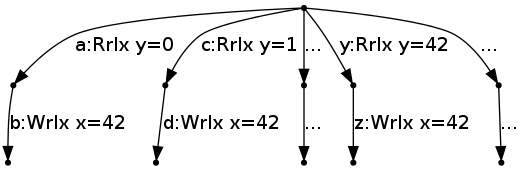Note that there is no memory state (see below for the treatment of memory state), as the thread does not have the information of which values it can read. Instead, the LTS of the thread has one branch for each value that might be read from `y`. The branches of the LTS are not the program-source conditional branches (the `if`s) of the thread; they are the choices of what values might be read by the thread. Within each of those, the program-source branches can be resolved, as there the previously read values are known.

From now on, to keep the diagrams readable, we illustrate only two branches: the one that reads 0, and the one that reads 42.

The state of the thread at any time in the base semantics is a node of the LTS (which we will highlight), initially the root node: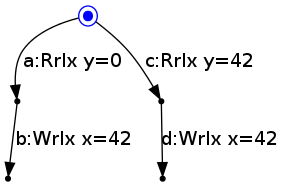In the base semantics, the state of the thread evolves by moving from the current node to another node by performing the action labelling an edge between them. For example, from the root node, Thread 2's state can move to the middle left node by reading 0 for `y`: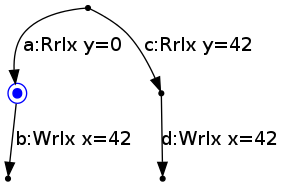Thread 2's state can then move to the bottom left node by writing 42 to `x`: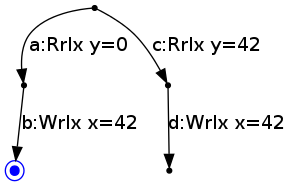Thread 2 then can not take any more actions.

## Derived semantics by example

Our derived semantics builds on top of the base semantics by adding the possibility of performing actions out of order.

Instead of taking states to be single nodes of the base LTS, a thread state in the derived semantics is a copy of the entire base LTS in which some edges are ticked, indicating that those actions have been performed, and some are unticked. The initial state is the one in which no edges are ticked. The transitions of the derived edge are labelled with the same actions as before but involve ticking a non-empty set of unticked edges.

For example, Thread 2 above, which in the derived LTS is initially in state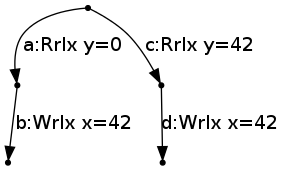with no ticks, can perform the same action as before, reading 0 for `y`, by transitioning to the state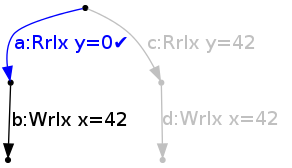It does this by ticking the (singleton) set of edges {a}. It can then transition to the state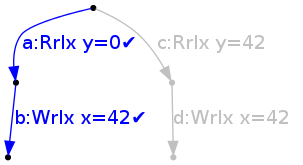by writing 42 to `x` and ticking {b}.

However, from the initial state, it can also do an out-of-order transition, writing 42 to `x`, and ticking the set of two actions {b,d}, to reach state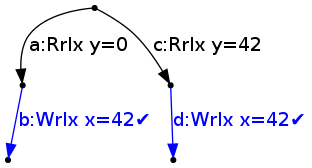This is allowed because in all branches that the thread can take, it will write 42 to `x` (action b on one branch and d on the other), which corresponds to the optimisation above. In other words, we can find a frontier across the tree with (loosely) that write present on all of them: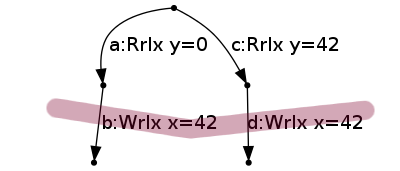It can then tick {a} by reading 0 for `y`, to reach state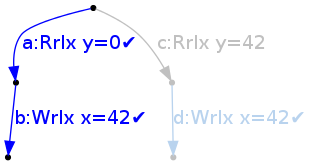It is now determined that the thread has gone down the a branch, so the branch starting at c is discarded. This discards the edge d, which had been ticked; this is desired behaviour: the fact that the `W x=42` was available in all branches made it possible to perform it before reading from `y`.

On the other hand, if we consider the thread

``````r2 = y;
if (r2 == 42) {
x = 42;
} else {
// nothing here
}``````

then the corresponding base LTS is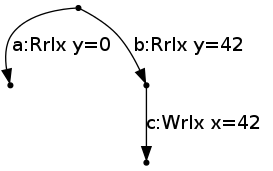and in that case, the write of 42 to `x` can not be performed right from the start, because it is not available in both branches. To be able to perform it, the program has to first go down branch b by reading 42 for `y`.

## Derived semantics: a more precise definition

More generally, a nonempty set E of edges can be ticked, performing the action a, iff

1. none of the edges E have already been ticked.

2. all the edges in E are labelled with action a.

3. all the (actions of the) edges in E can be performed. In particular, none of them are program-order-after an action to the same location that has not yet been ticked (and hence should be blocked by coherence), and none of them are program-order-after a fence that has not been ticked.

4. the edges in E "form a frontier", meaning that E corresponds to a memory action that can be performed in all "non-discarded" branches of the program (intuitively, branches in which we do not know yet that the program is not going to go into; formally, branches such that no edge has a ticked sibling edge).

The formal definition is expressed in Lem code, and we have used that to build a command-line tool allowing interactive exploration of small examples.

## Checking the other examples from notes42:

• 1 Example LB (language must allow). Allowed, as the writes of `x` and `y` can both be done first, out-of-order.

• 2 Example LB+datas (language could and should forbid).

``````r1 = x // reads 42;
y = r1;
-------
r2 = y // reads 42;
x = r2;``````

Forbidden: the base LTS of Thread 2 is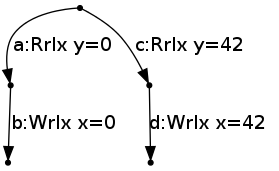and it is not possible to perform `W x=42` (or `W x=0`) from the initial state, as it is not available (or even present) in both branches.

• 3 Example LB+ctrl+data+po (language must allow). Allowed, as the write of `x=42` on the second thread can be done first.

• 4 Example LB+ctrl+data+ctrl-double (language must allow). This was our introductory example. It is allowed, similarly to 3 (as one would want), as the write of `x=42` on the second thread can be done first.

• 5 Example LB+ctrl+data+ctrl-single (language could and should forbid). Forbidden, as neither write can be done out-of-order.

• 6 Dependencies via other compilation units. The example is:

``````// in one compilation unit
void f(int ra, int*rb) {
if (ra==42)
*rb=42;
else
*rb=42;
}

// in another compilation unit
r1 = x; // reads 42
f(r1,&r2);
y = r2;
-------------------
r3 = y; // reads 42
f(r3,&r4);
x = r4;``````

Our current prototype doesn't support functions or pointers, but just looking at the body of `f`, it allows it to behave like

``````*rb=42;
if (ra==42) {} else {}``````

which makes it possible to perform `f` and the writes of both threads before the reads, which makes it possible for the reads of `x` and `y` to read 42.

## Further examples

To illustrate the coherence condition, consider a thread

``````x = 1;
x = 2;``````

which yields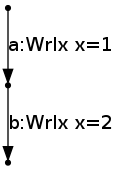Because of coherence, the edges {b} can not be ticked (performing `W x=2`) initially, because b is after a non-ticked edge (a) to the same location (`x`). Once the edges {a} have been ticked, the edges {b} can be ticked, performing `W x=2`.

Similarly, with fences:

``````x = 1;
fence();
y = 1;``````

yields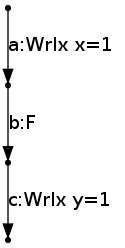and the edges {c} can not be ticked from the beginning, because c is after an unticked fence. However, it is possible to tick the edges {a}, performing `W x=1`, then the edges in {b}, performing `F`, and only then the edges in {c}, performing `W y=1`.

Out-of-order transitions can be further in the "future", e.g. for this thread:

``````r1 = x;
if (r1 == 0) {
y = 1;
r2 = z;
} else {
y = 2;
r2 = z;
}``````

which yields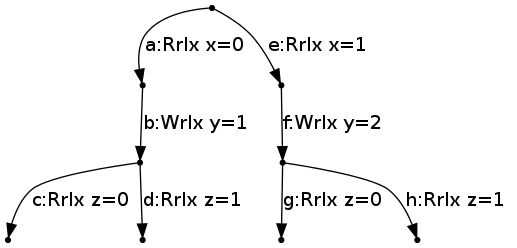neither {b} nor {f} can be ticked, but {a}, {e}, {c,g}, {d,h} can be ticked.

Once {a} is ticked, {b}, {c}, and {d} can be ticked.

## Non-multi-copy-atomic memory

Given this thread-local semantics, to define the behaviour of a complete multi-threaded program, we also have to define how the threads interact via the memory. In particular, their interaction should not be multiple-copy-atomic, as the POWER and ARM architectures are not. For this, we envisage using basically the same "storage subsystem" as the operational POWER memory model by Sarkar et al. `http://www.cl.cam.ac.uk/~pes20/ppc-supplemental/index.html`.

Note that in our prototype tool we currently use an SC storage subsystem (for two-thread examples it makes no difference).

## Discussion

### Value-range analysis

Note that this scheme is incompatible with value-range analysis for shared variables. Consider for example the thread

``````r2 = y;
if (r2 == 42) {
x = 42;
} else {
// nothing here
}``````

If value-range analysis determines that in fact the only value that `y` can contain is 42, then it can perform the write to `x` out of order, whereas our thread-local semantics does not allow that. Note that this applies to all shared memory accesses, even non-atomic ones, and even accesses for which scoping ensures that all accesses to the same location are guaranteed to arise from the same function or compilation unit.

### Status of variables

Our semantics (our toy source language, actually, but it reflects in the semantics) assumes two distinct classes of variables: "register" variables (`r1`, `r2`, ...), which are used for thread-local data flow, and shared-memory variables (`x`, `y`, ...) , which are used for inter-thread communication. However, C does not have two distinct classes, so it is not directly apparent whether a variable is thread-local. It might still be possible to separate the two by a suitable fixed-point construction in a way that satisfactarily approximates the compiler behaviour.

### Memory action elimination and introduction

Intuitively our semantics can be viewed as an operational version of the trace-set-semantics view of reorderings as used by Sevcik, Zappa Nardelli, etc., and this begs the question of whether that can be made precise and extended to cover eliminations and introductions (both of which might enable more frontiers to be constructed and hence more reordering). Looking through the cases of Definition 1 of Safe Optimisations for Shared-Memory Concurrent Programs. Jaroslav Sevcik, PLDI 2011, we can sketch possible adaptations as follows (this is speculative; none of them have been worked out in detail):

``````r1 = x;
y = r1;
---------------
r2 = y;
if (r2 == 1) {
r3 = y;
x = r3;
} else {
x = 1;
}``````

then, assuming `y` is not volatile and the read is not `ACCESS_ONCE`, it is resonable to eliminate `r3 = y`, and replace it by `r3 = r2` (thanks to Jaroslav Sevcik for highlighting this example). Currently, this is not considered by our semantics. In a way, all our accesses are `ACCESS_ONCE`.

Intuitively, it seems plausible that this could be dealt with by nondeterministically discarding (or `anti-ticking') paths in which consecutive reads of the same location see different values.

• Irrelevant read elimination: It may be that this is harmless (in the sense that handling it explicitly would not permit more frontiers to be created, so not needed for allowing all the desired out-of-order observable behaviours).

• Redundant write after read elimination, Redundant overwritten write: Likewise harmless?

• redundant last write, redundant release, redundant external action: we skip all this end-of-trace clauses

Redundant read introduction might be handled by taking a more liberal notion of frontier, allowing out of order reads even if they don't occur on all paths.

### Undefined behaviour

How does this interact with the fact that C has many sources of undefined behaviour? For those which are thread-local (i.e., not data races), one could simply regard any path that reaches such a point to be irrelevant for frontier calculations. This would require an operational definition of these sources of undefined behaviour in which one can see them occuring at particular points in execution time, with an undefined-behaviour-free prefix. But that's no problem -- we can do it.

### Non-atomics

The presentation above focused on relaxed accesses. Our semantics also includes non-atomic accesses, which differ in that non-atomic accesses are not performed out-of-order. This is for modeling purposes; the point is that if there is a data race on non-atomics performed out-of-order, then there is one with non-atomics performed in order (note: this has to be established); on the other hand, if there is no data race, then the fact that the non-atomic accesses are performed out-of-order can not be observed.

Note that we do not allow value-range analysis or elimination/introduction even for shared-memory non-atomics.